# 标识符的作用域

## 一、函数原型作用域

#### 实验1：在函数内声明一些变量，在函数外使用，然后观察编译器的反应，以及如何解决。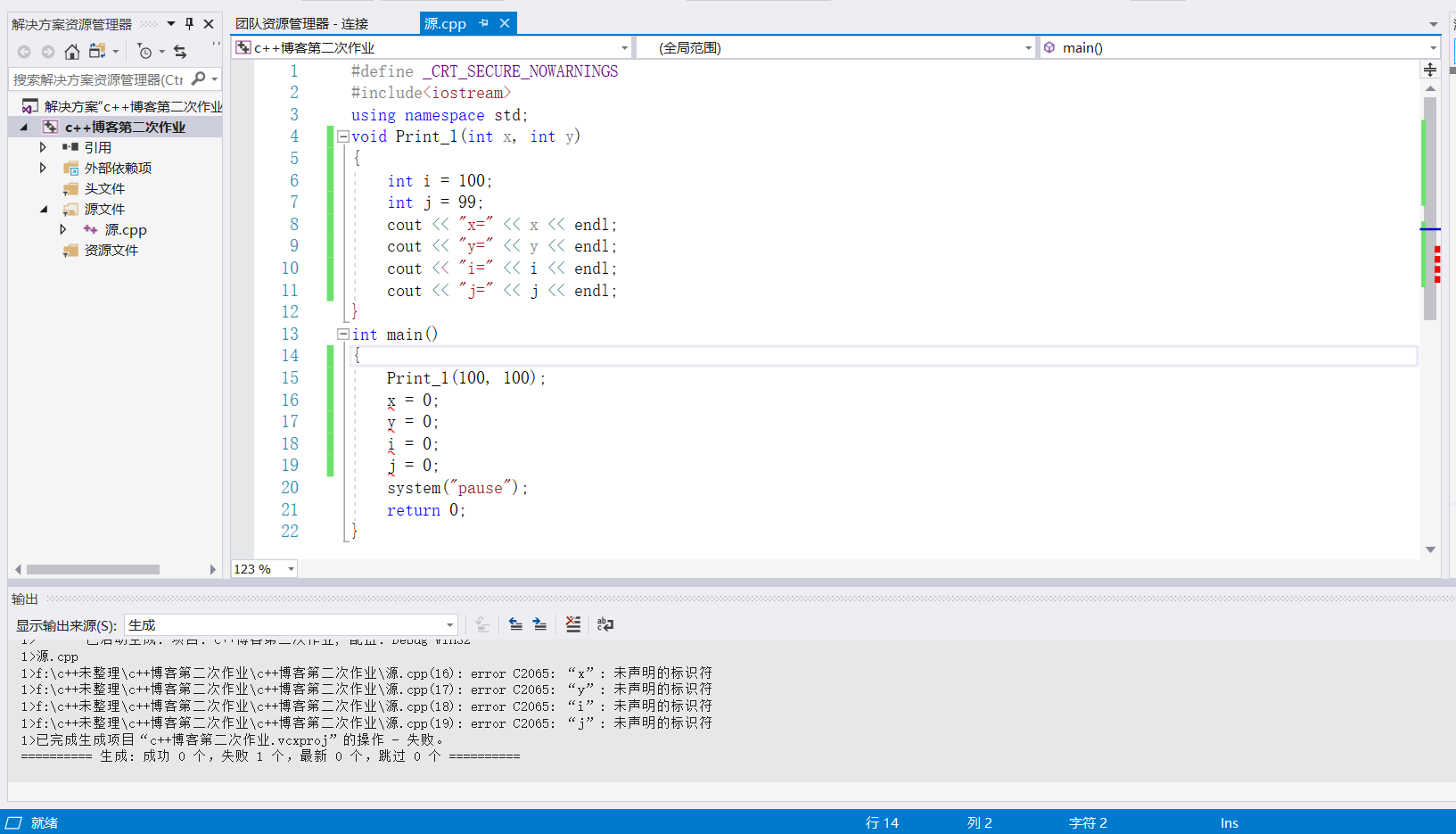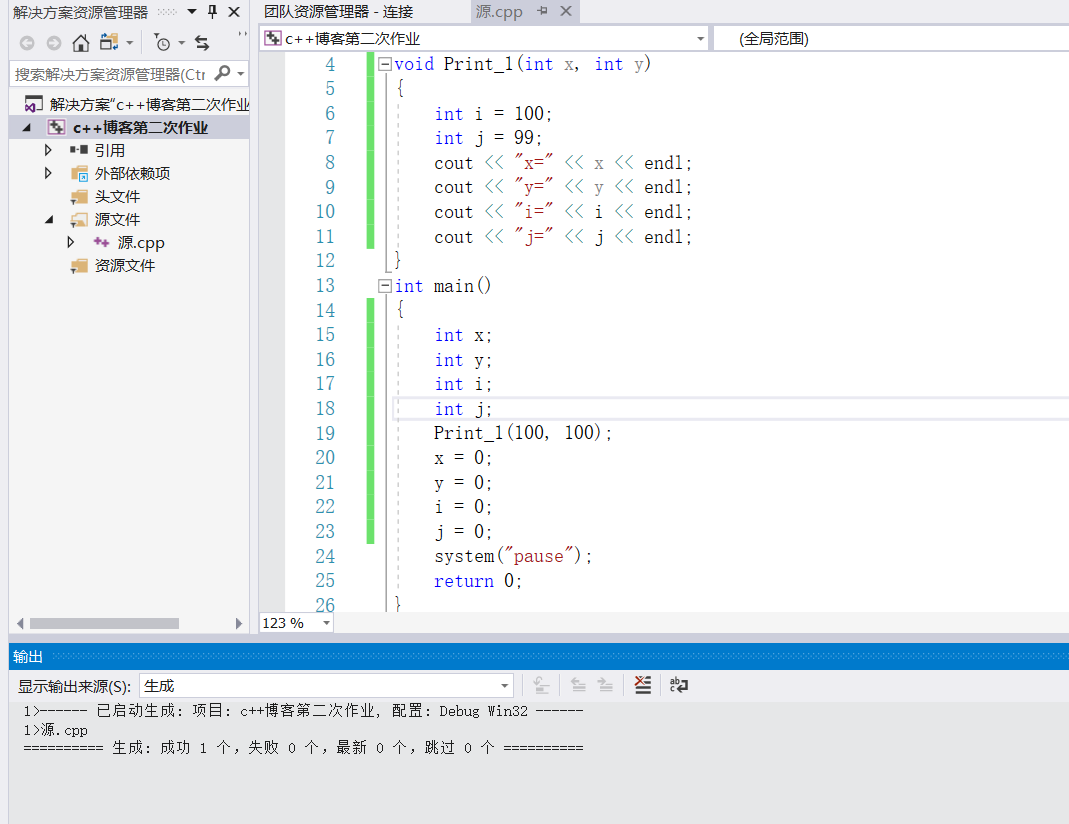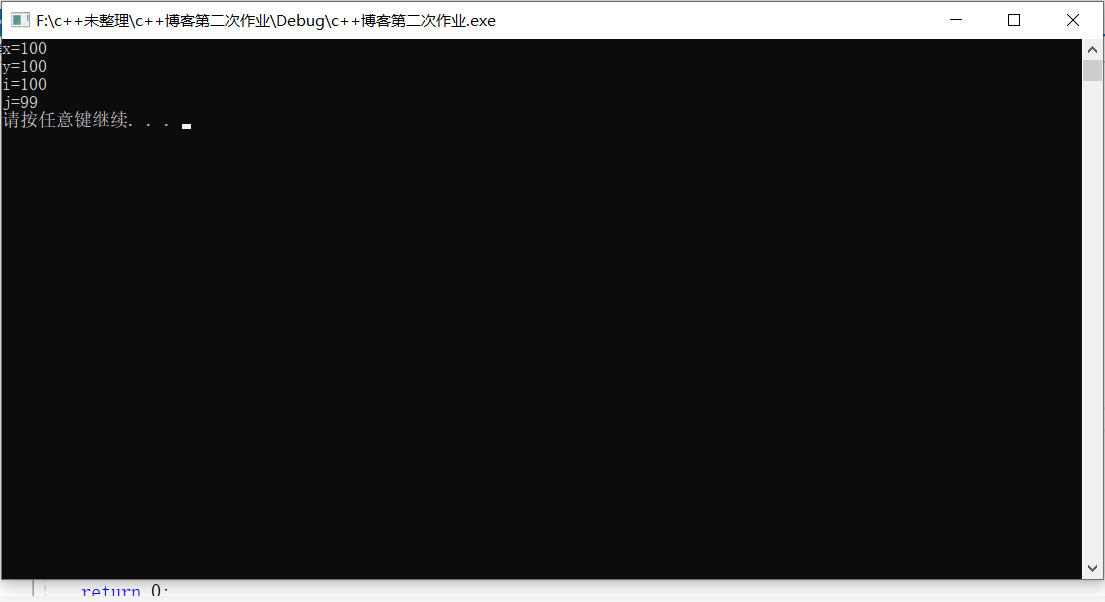## 二、局部作用域（块作用域）

#### 实验2：在块外面声明变量，然后再在块里面声明同样标识符的变量，并分别在块内和块外使用cout语句将变量的值输出到屏幕，查看结果，并尝试去查找原因。

#define _CRT_SECURE_NOWARNINGS
#include<iostream>
using namespace std;
int main()
{
int flag=1;
int i = 2;
int j = 3;
if (1)
{
int flag = 78;
cout << "块内flag=" << flag << endl;
if (1)
{
int i = 28;
cout << "块内i="<<i << endl;
if (1)
{
int j = 45;
cout << "块内j=" << j << endl;
}
cout << "块外j=" << j << endl;
}
cout << "块外i=" << i << endl;
}
cout << "块外flag=" <<flag<<endl;
system("pause");
return 0;
}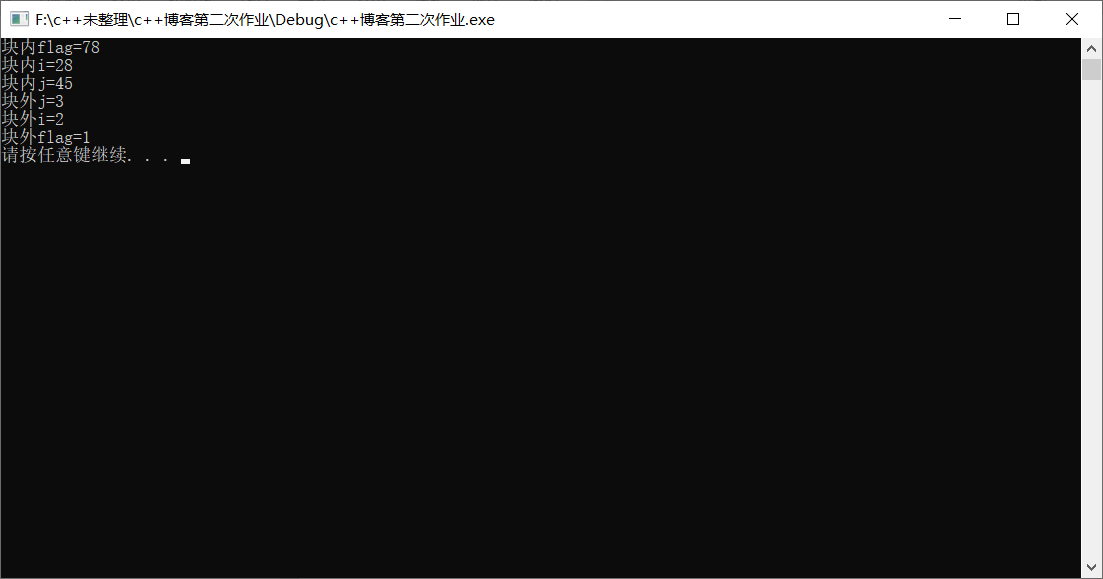## 三、类作用域

#### 实验3：写一个名为Circle的类，有成员R,一个计算面积的成员函数，一个构造函数。


#define _CRT_SECURE_NOWARNINGS
#include<iostream>
using namespace std;
#define Pi 3.14
class Circle {
public:
Circle(float R)
{
}
double getArea()
{
}
private:
};
int main()
{
float R;
cout << "请输入圆的半径" << endl;
cin >> R;
Circle MyCircle(R);
cout << "该圆的面积为" << MyCircle.getArea() << endl;
system("pause");
return 0;
}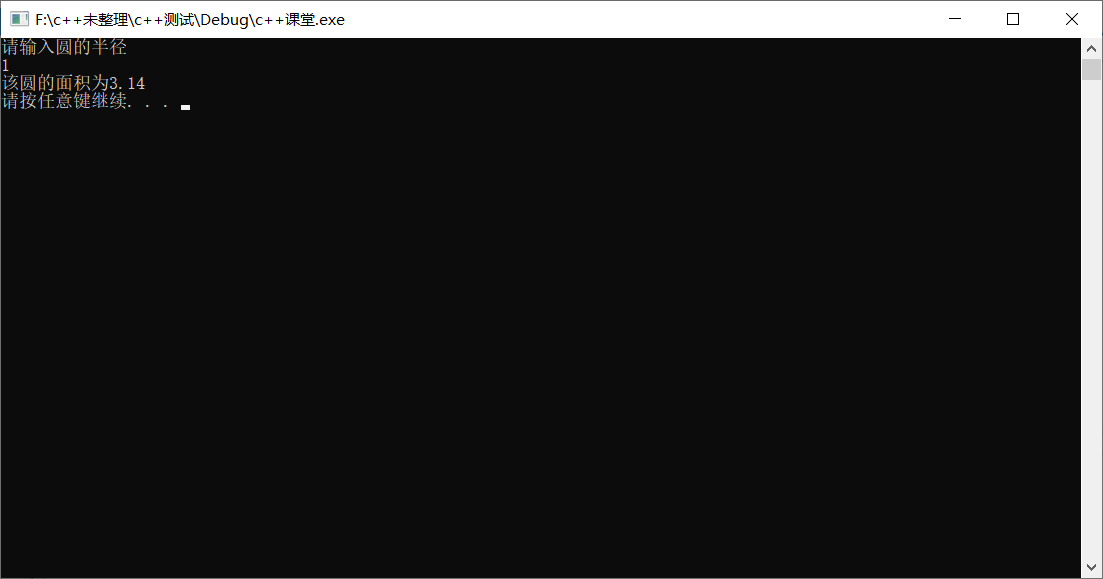## 四、命名空间作用域

#### “一个命名空间确定了一个命名空间作用域，凡是在该命名空间之内声明的、不属于前面所述各个作用域的标识符，都属于该命名空间作用域。”

using namespace std;


#### 实验4：将一个类的声明写在一个命名空间里，并将这个命名空间写在一个头文件中，并在主函数中对其中的标识符进行操作。

myspace.h

//myspace.h
#define Pi 3.14
namespace myspace
{
class Circle {
public:
Circle(float R);
double getArea();
private:
};
}



//类的函数.cpp
#include"myspace.h"
using namespace myspace;
Circle::Circle(float R)
{
}
double Circle::getArea()
{
}


// 源.cpp
#define _CRT_SECURE_NOWARNINGS
#include<iostream>
#include"myspace.h"
using namespace std;
using namespace myspace;
int main()
{
Circle circle(1);
cout << "圆的面积为：" << circle.getArea()<<endl;;
system("pause");
return 0;
}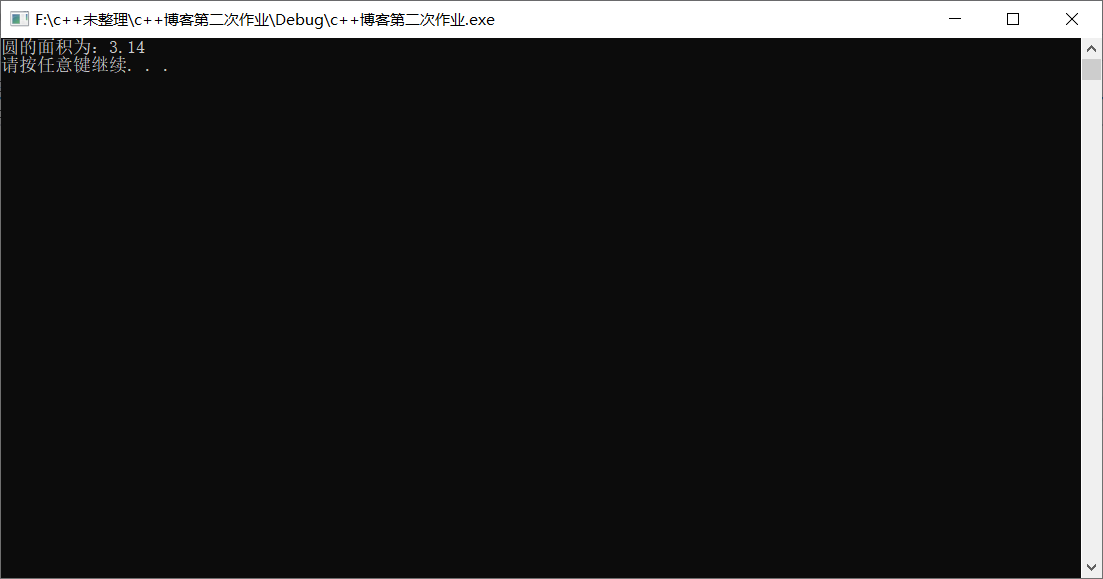posted on 2019-09-27 14:09  Tlyh  阅读(683)  评论(0编辑  收藏  举报